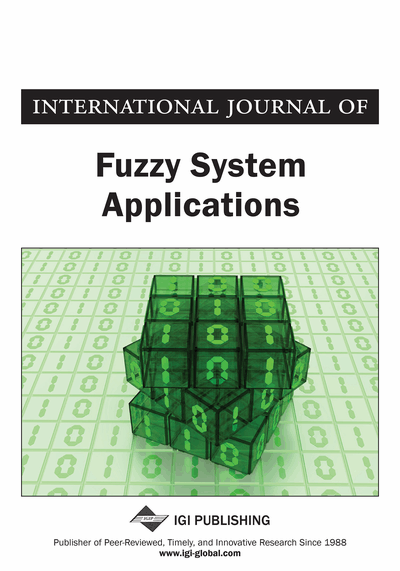# A Resource-Constrained Project Scheduling Problem with Fuzzy Activity Times

Hossein Zoulfaghari (Department of Industrial Engineering, Isfahan University of Technology, Isfahan, Iran), Javad Nematian (Department of Industrial Engineering, University of Tabriz, Tabriz, Iran), and Amir Abbas Kanani Nezhad (Department of Industrial Engineering, Khaje Nasir University, Tehran, Iran)
DOI: 10.4018/IJFSA.2016100101
Available
\$29.50
No Current Special Offers

## Abstract

This paper is about the Resource-Constrained Project Scheduling Problem) RCPSP) which is one of the most important problems in last three decades and many researchers have paid attention to it and have reached useful results. In this paper, to cope with uncertainty issue, the RCPSP is studied under fuzzy environment where activity times are assumed to be fuzzy numbers. For this problem with fuzzy numbers as activity times, a linear mathematical programming model is presented. The objective function of the model is minimizing the completion time of project. Since the activity times are fuzzy numbers, finish time is also a fuzzy number. Hence, the model is transformed to a crisp multi-objective linear programming model. To illustrate the solution method, a numerical example is solved under both fuzzy and crisp environment and the results are compared. To prove the efficiency of the proposed method the results of the proposed solution method, some benchmark problems obtained from PSPLIB are utilized.
Article Preview
Top

## 1. Introduction

The Resource-Constrained Project Scheduling Problem (RCPSP) is one of the problems in scheduling and control project which includes allocation of limited resources to activities with effective methods. This characteristic caused the RCPSP to be categorized as NP-Hard problems (Demeulemeester, E. & Herroelen, W. 2004). Not only exact solution procedures but also many heuristic methods have been proposed to solve RCPSP. (Kolisch et al. 2000) provided detailed history of the work conducted in this area. Being an NP-hard problem, (Alcaraz et al. 2001) mentioned that the optimal solution can be achieved by means of exact solution procedures but only in small projects, usually with less than 60 activities. So, meta-heuristic methods such as Tabu Search (Baar, T. et al. 1997; Pirson, E. et al. 1994), Simulated Annealing (Boctor, F.F. 1996; Bouleiman, K. & Lecocq, H. 2003) and Genetic Algorithms (Alcaraz J. & Maroto, C., 2001; Zoulfaghari, H. et al., 2013) are presented to solve large scale problems. However, in all of these cases, these problems have been modeled and solved in crisp environment. But, providing a precise estimation for the activity times and amount of needed resources is not possible in this situation, which causes imprecision in the data. In order to overcome this problem, application of fuzzy set approach for modeling may be much useful. The use of fuzzy numbers for scheduling was started by Prade (Prade, H., 1979). Many other researchers followed the same work which was done by Prade. For instance, Chang et al. (1995), Yao and Lin (2000), McCahon (1993) Chanas and Zielin´ski (2002), Kuchta (2001), Dubois et al. (2003) and Nematian et al. (2010) have done some researches in this area. Main scope in this paper is solving the RCPSP in fuzzy environment and its results will be investigated on a set of bench-mark problems. For scheduling a project, the formulation by Alvarez-Valdés and Tamarit (1989) is presented. Furthermore, the activity duration times are considered as fuzzy numbers. To solve the model with these assumptions, it changes to a crisp multi-objective linear programing problem. To solve this problem, Global Criterion Method (Chang et al. 2009; Kaufman et al. 1988; Buckly et al. 2000; Alvarez-Valdes et al. 1989; Bhattacharyya et al. 2011) is used. The rest of the paper is organized as follows. In section 2, some basic fuzzy relations which are used in converting fuzzy environment to crisp one are explained. In section 3, the linear programming model of RCPSP under fuzzy environment is presented and its conversion to a multi-objective crisp model is described. Global criterion method used to solve multi-objective model is elucidated in section 4. Our computational results are reported in section 5. Conclusions and suggestions for further research are discussed in last section.

## Complete Article List

Search this Journal:
Reset
Volume 12: 1 Issue (2023)
Volume 11: 4 Issues (2022)
Volume 10: 4 Issues (2021)
Volume 9: 4 Issues (2020)
Volume 8: 4 Issues (2019)
Volume 7: 4 Issues (2018)
Volume 6: 4 Issues (2017)
Volume 5: 4 Issues (2016)
Volume 4: 4 Issues (2015)
Volume 3: 4 Issues (2013)
Volume 2: 4 Issues (2012)
Volume 1: 4 Issues (2011)
View Complete Journal Contents Listing